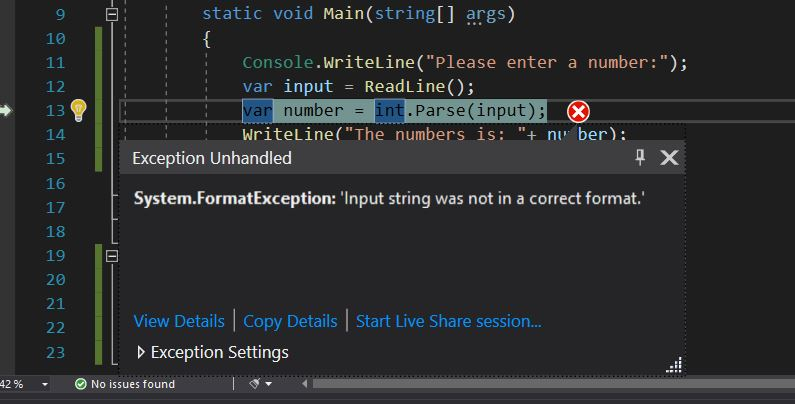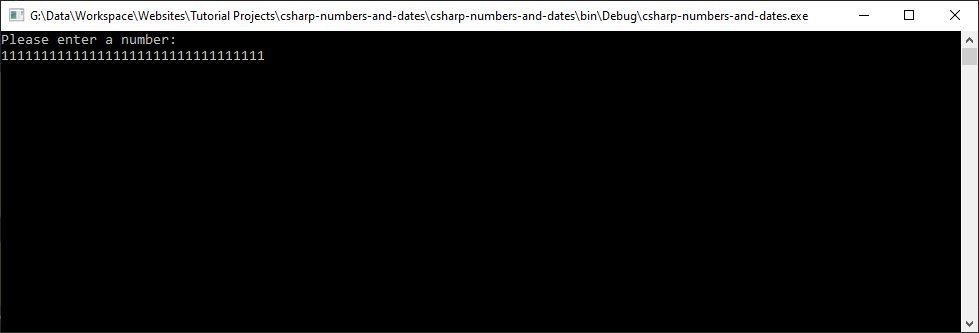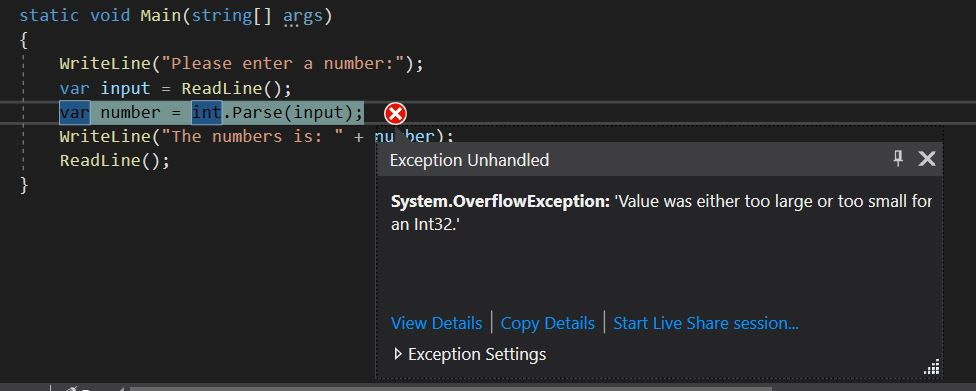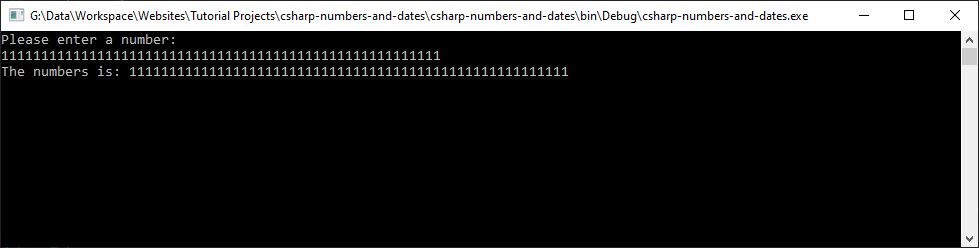Whether you’re still learning C# or you already have some experience, there will always be gaps in your knowledge, places where you are clueless as to your ignorance.

In the series of articles in C# Tips I will provide a whole host of useful information about the sometimes underused or unknown features of both C# and .NET.

In the previous article, we learned some tips for working with c# strings and formatting. In this article, we’re going to be covering some tips for working with numbers and dates.

## Parsing Strings into Numbers with the NumberStyles Enumeration

We can see in the user instructions here we’re asking the user to input a number. We’re then just reading the input from the console and using the int.Parse method to convert that string into an int.

If we run the above program, enter a number and hit enter we will get the following output:

Now, what if we enter a number but using thousands separator like this `1,000`. In that case, the above program will give the following exception.Now the thing is when we have some string input and we want to convert it into a numerical representation we can control how the parsing takes place. To control the parsing, we can use an overload of the Parse method that allows us to specify one of the NumberStyles enumeration values like this:

The above program generates the following result:

NumberStyles has the whole host of different options to fine‑tune the parsing, such as allowing a hex specifier, allowing parentheses to represent negative values, allowing thousands separators, and so on.

So when the int.Parse method gets executed, we’re allowed to specify thousands separators.

We can also combine NumberStyles, we can create our own custom versions. To specify custom formatting specifications, we simply use the bitwise OR operator and then specify a different NumberStyles value.

So in our case, perhaps we wanted to say that will allow leading whitespace along with trailing whitespace. We can create this custom specification like this:

The above program generates the following result:

## Preventing Ambiguous DateTime Parsing and Mis-parsing

We can see in the user instructions here we’re asking the user to input a date. We’re then just reading the input from the console and using the DateTime.Parse method to convert that string into a `DateTime`.

The above program generates the following result:

But what if I want to mention the month before the day, in that case, the method will missparse the datetime. We can help prevent mis‑parsing of dates by specifying the exact format that we expect.

We’re instead going to call DateTime.ParseExact.

This method allows us to specify the string we want to parse. In our case, it’s the input from the console. We can then specify in the format that we expect the date to be in. So, I wanted to allow the month to be specified first. We can use the format months, days, and a four-digit year.

The above program generates the following result:

## Parsing DateTimes with the DateTimeStyles Enumeration

Just as we used the NumberStyles enumeration to help control formatting, so too can we use the DateStyles enumeration. Just as a quick example here, I’m going to use some hardcoded values.

Let’s take a look at the d2 variable.

In this example, we’re using the DateTimeStyles.AssumeUniversal. If we look at the output, we’ve got the 1st of December 2000, but notice this time that the time component has been set to `05:30` AM. If we compare this to d1, notice the time component is midnight. When we use DateTimeStyles.AssumeUniversal, it’s going to assume that the date is a UTC date. Because I’m recording this in India and India is `05:30` hours ahead of GMT, we get `05:30` hours added on to midnight, which is 5:30 AM.

Let’s take a look next at d3.

Here we’re specifying DateTimeStyles.AssumeLocal. If I look at the output, this is essentially the same as d1. We’ve got the time component set to midnight.

But what happens if we’re parsing a string that just contains a time component? Let’s take a look at this d4.

We haven’t specified a DateTimeStyle, so this is going to default to `DateTimeStyles.None`. Notice here that we’ve successfully got 1:30 PM parsed from this 24 hour 13:30:00 string, but the date component of this time has been set to today’s date(the date I ran the program on my machine), the 31st of March 2020.

If we want to parse time, but we don’t want to automatically add the current date, we can use the DateTimeStyles.NoCurrentDateDefault. And that’s what we’ve done for d5.

Notice that we’ve successfully parsed 1:30 PM. or 13:30:00 in the 24‑hour clock, but unlike with d4, the date component has been set to DateTime.Min, which is the first of the first 0001. So, in effect, we’ve created a DateTime object with time, but no specific date.

So that’s a couple of different ways we can control the parsing of dates and times, one by using the ParseExact method and specifying the expected format, and the second by making use of one of the DateTimeStyles enumeration values.

## Representing Arbitrarily Large Integer Values

If we go and run the above program and try and specify a number that’s greater than the maximum value an int can hold and hit EnterWe will get OverflowException like this:If you want to represent integer values that are greater than the standard data types, you can make use of the BigInteger class. To get access to the BigInteger class, we need to add a using directive to `System.Numerics`.

Just as we did with the int version, we can use the `BigInteger.Parse` method. This is a static method of the BigInteger class, and we can use this to parse the string input into a BigInteger value.If we run the app this time and once again enter the same value and hit Enter, notice this time we don’t get an exception. That’s because now we’re representing the number as a BigInteger, and a BigInteger instance can hold arbitrarily large integer values.

One thing to bear in mind if you’re going to use the BigInteger class is that because it can hold an arbitrarily large value, you may risk getting an out-of-memory exception.

Also, because BigInteger instances are immutable, anytime you, for example, add value to an existing BigInteger, you’ll get a new BigInteger assigned. Once again, if you’re working with massive values, you may risk out-of-memory exceptions.

Just as with the standard data types, you can write code such as increment, decrement, multiplication, and the other standard operators.

However, one thing to bear in mind is that the methods in the Math class, such as Math.Max, don’t support big integers.

So if you wanted to use Math.Max with big integers, you can instead use one of the static methods of the BigInteger class itself to replicate the functionality from the Math class.

So that’s how we can use the BigInteger class to represent arbitrarily large integer values.

## Creating Random Numbers

The first method we’re going to look at to create random numbers makes use of the Random class. The Random class exists in the System namespace, and we’ve already got a using directive for System.

The Random’s Next method generates a non-negative random integer. There’s a couple of overloads of the Next method, one of which allows us to specify an integer value, and this overload will limit the maximum random number that’s returned like this:

The number that we supply here in this example, 128, will be one more than the maximum number returned. So, for example, here, the maximum random number that we would ever get is 127.

There is another overload of the Next method which allows us to specify the range of random values that we want.

Here we’re saying the minimum random number is -100, and the maximum is 100. Unlike the previous two versions of the Next method, this method can return negative integers. One thing to bear in mind when using this method is that the maximum we specified as 100 here is once again one more than the actual maximum that we could get. So we’ve specified a maximum of 100. The most we’d ever get is 99.

In addition to generating integer values, we can also fill a byte array with a random selection of bytes. So, for example, if the byte array that we passed into it had four elements, we could get a resulting array populated with random numbers like this,

We can also generate random floating‑point numbers by using the NextDouble method. This method will return a random floating‑point number that is greater than or equal to 0.0 and less than 1.0. So, for example, here we could get a resulting floating‑point number such as this,

There are a few things to note when making use of the Random class, however. When we use this constructor that doesn’t take a parameter, it’s going to use the system clock as the seed value to generate the sequence of random numbers. If we want to, however, we can supply our own seed value.

Here we’re using a seed value of 128, so the random numbers generated from this `random` instance will be based on a seed value of 128. Different random instances with different seed values will produce different sequences of random numbers.

Another thing to bear in mind when using the Random class is that if you create two instances close together and don’t specify a seed value, they may actually generate identical sets of random numbers. That’s because, once again, if we don’t specify an explicit seed value, the system clock will be used. So if we create two instances so close together that the system clock is the same, then we’ll get identical seed values used.

It’s better to reuse a single random instance rather than generating a new instance of the Random class for every number you want to generate. However, if you do use a single random instance, you should also note that random objects are not thread safe.

So if you’re working in a multi-threaded environment, you’ll need to add some manual locking code around the random object. Another thing to bear in mind is that the actual internal implementation of the random number generator inside the Random class may change. So, for example, if we specify a seed value, for example, 128, we may get one sequence of random numbers generated. But in the future, if the .NET Framework changes, the same seed value of 128 may generate different sequences of random numbers.

One major thing to be aware of is that numbers generated from a random instance are not completely random; however, the documentation states that they’re sufficiently random for practical purposes. One purpose that the Random class is not suitable for, however, is for creating cryptographically secure random passwords. If you want to generate cryptographically secure random numbers, you should instead make use of the RNGCryptoServiceProvider class. Let’s take a look at this next.

## Creating Cryptographically Secure Random Numbers

To do this, we’re going to use the RNGCryptoServiceProvider. This class exists in the System.Security.Cryptography namespace, so we’ll add a using directive to that namespace.

As `RNGCryptoServiceProvider` class implements IDisposable, we wrapped it in a using statement. The way this class works is that it will fill an array of bytes with random values. We can then convert this array of bytes to the data type that we want.

In our case, this is going to be an int. Because we want to generate an int, we’re going to need 4 bytes of data.

Now we’ve got an array of bytes that we can fill with random values, we can call the RNGCryptoServiceProviders GetBytes method and pass in the array that we want to fill. In this case, it’s the 4‑byte array, randomBytes.

So now we’ve got an array of bytes containing cryptographically secure random byte values, we need to convert this array to an int. And to convert this array of 4 bytes into an int., we’re going to be using the BitConverter class.

That’s how you can create a cryptographically secure random number using the RNGCryptoServiceProvider class.

## Generating Sequences of Integer Values

For the final tip in this article, let’s see how we can easily generate a sequence of integer values.

From the code in the above program, you can conclude that we are generating a sequence of numbers from 0 to 9. However, we can simplify this code quite a lot and get rid of the for loop completely by making use of the Enumerable class.

First off, we’re going to make use of the Enumerable class, and to get access to this class, we need to add a using directive to the System.Linq namespace. We can then call the static Range method. This allows us to generate an IEnumerable of ints by specifying the start value and the number of elements that we want.

So in our case, we want to start the sequence at 0, and we want 10 elements. You can also use LINQ here to do some more advanced generation. For example, we could use the Select method and say x goes to `x*2`.

Here we still have the sequence 0 through 9 generated, but because of the Select, each element was then doubled, so now we get the values 0,2,4,6,8,10,12,14,16,18.

So that’s how we can use the static Range method of the Enumerable class to generate sequences of integer values.

## Summary

So that brings us to the end of this article. In this article, we started by learning how we can control the parsing of strings into numbers by making use of the NumberStyles enumeration, such as the ability to specify that numbers could contain a trailing sign.

We learned how we can help prevent the mis‑parsing of date strings into DateTime objects by making use of the ParseExact method and specifying the exact format that we expect.

We also learned that we can control DateTime parsing by making use of the DateTimeStyles enumeration, such as preventing the current date being used if we’re parsing a time‑only component.

We learned that we can represent arbitrarily large integer values by using the BigInteger class, but when we do this, we should take care not to get out‑of‑memory exceptions.

We then looked at a couple of ways of generating random numbers, the first using the Random class and the second using the RNGCryptoServiceProvider.

Finally, we looked at a quick way of generating sequences of integer values by using the static Range method on the Enumerable class. That’s it for now, join me in the next article by subscribing to my newsletter using the form below.